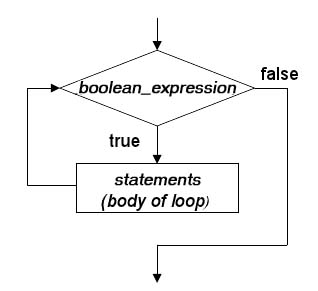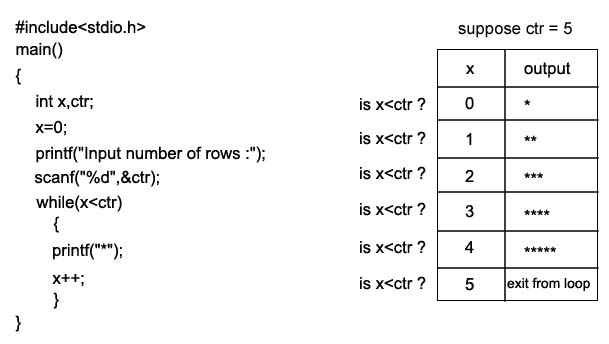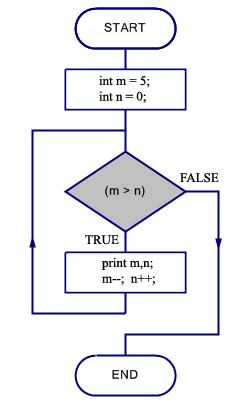## Description

The most basic loop in C is the while loop and it is used is to repeat a block of code. A while loop has one control expression (a specific condition), and executes as long as the given expression is true. Here is the syntax :

## Syntax

while (condition)

{

statement(s);

}

In while loop first the condition (boolean expression) is tested; if it is false the loop is finished without executing the statement(s). If the condition is true, then the statements are executed and the loop executes again and again until the condition is false.
If there is only one statement, the braces may be omitted; however, it is good practice to always include the braces.

See the flowchart :## A Simple While Loop Example -1

The following program prints n asterisks.## Example - 2

The following program prints two numbers.

1. #include <stdio.h>
2. main()
3. {
4. int m = 5;
5. int n = 0;
6. while (m > n)
7. {
8. printf("m = %d n = %d\n",m,n );
9. m--;
10. n++;
11. }
12. }

m = 5 n = 0
m = 4 n = 1
m = 3 n = 2

## Explanation

The loop will terminate when m becomes equal to or lesser than n, hence m <= n is the terminating condition of the while loop.

The loop has executed 3 times.

The condition m>n has checked 4 times.

First time : m = 5 and n = 0, this time the condition is true, so loop is executed.
Second time : m = 4, and n = 1. Again the condition is true, so loop is executed.
Third time : m = 3 and n = 2. Again the condition is true so loop is executed.
Fourth time : m = 2 and n = 3. Now the condition is false, hence loop is not executed.
Therefore this is the last time the condition m>n is checked in the program.

Here is the flowchart of the above programÃ¯Â»Â¿

source:http://www.w3resource.com/c-programming/c-while-loop.php